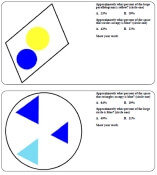edHelper.com Math Worksheets Percents

Percents and Estimation:  Estimate and Percents Shape PuzzleEstimate and Percents Shape Puzzle (requires knowledge of percents - grades 6+)

Write the percent of the shaded portion in each grid
Write each fraction as a percent
Write each decimal as a percent
Write each ratio as a percent
Write each fraction, decimal, or ratio as a percent
Write the percent as a fraction
Write the percent as a decimal
Write the percent as a ratio
Algebra: Fill in the missing number
Order 3 numbers from least to greatest
Order 3 numbers from greatest to least
Order 6 numbers from least to greatest
Order 6 numbers from greatest to least
Find 10% of each number
Find the percent of each number (multiples of 5%)
Find the percent of each number
Percent of numbers: Find the missing number
Open-ended questions
Circle graphs
Percents Mixed Review

Write percent as fraction
Write percent as decimal
Write decimal as percent
Write fraction as percent
Write fraction as percent (including irrational fractions)
Fill in missing value (2 items)
Fraction, decimal, and percent table
Percent of a number
Solve for percent of a number
Solve for percent change
Simple Interest (whole years and whole interest rates only)
Simple Interest
Percents Review Printable
Word Problems
General Percents Word Problems
Biology
Geology
Physics
Chemistry
Sports
Percents Word Problems Mixed Review

Writing Fractions as Percents
Writing Decimals as Percents
Comparisons Between Fractions, Percents, and Decimals
Percent of a Number
Percent of a Number (Much More Difficult)
Store Discounts and Taxes
All 1 year, only calculate Interest
All 1 year, calculate interest and total payments
Fractional Years and Decimal Rates
Less than one year (30, 90, .. 300 days)
Mixed Table with All of the Above!
Interest Word Problems (Difficult)

Percents Word Problem Worksheet
For best results, pick multiple options.
For example, you will not find division problems in the grade 1 material.
Select the skills to include:
 Addition Subtraction Multiplication Division Time Decimals Fractions Percents and Ratios